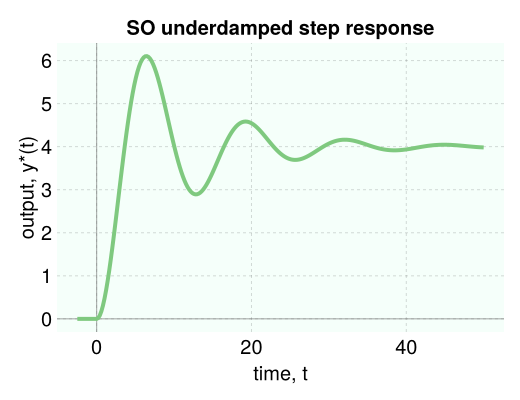# Controlz.jl

Controlz.jl is a Julia package to analyze and simulate process dynamics and control systems using transfer function representations.

for example, to simulate the unit step response of a second-order, underdamped system characterized by the transfer function

$$$g(s) = \dfrac{4}{4s^2 + 0.8s +1}$$$

the output $Y(s)$ follows from $g(s)U(s)$, where $U(s)$ is the input.

using Controlz

g = 4 / (4 * s ^ 2 + 0.8 * s + 1) # construct transfer function
U = 1 / s                         # unit step input, U(s)
Y = g * U                         # system output, Y(s)

data = simulate(Y, 50.0)          # simulate until t = 50

viz_response(data, plot_title="SO underdamped step response")# install the Controlz.jl package in Julia

• in the Julia REPL: go into package mode by typing ]. then add Controlz. then Backspace to exit package mode.
• in a Jupyter or Pluto Notebook: using Pkg; Pkg.add("Controlz"). (this way also works in the REPL)

for visualizations, Controlz.jl relies on PyPlot.jl, a Julia interface to matplotlib in Python. see here if you have trouble installing PyPlot.jl.